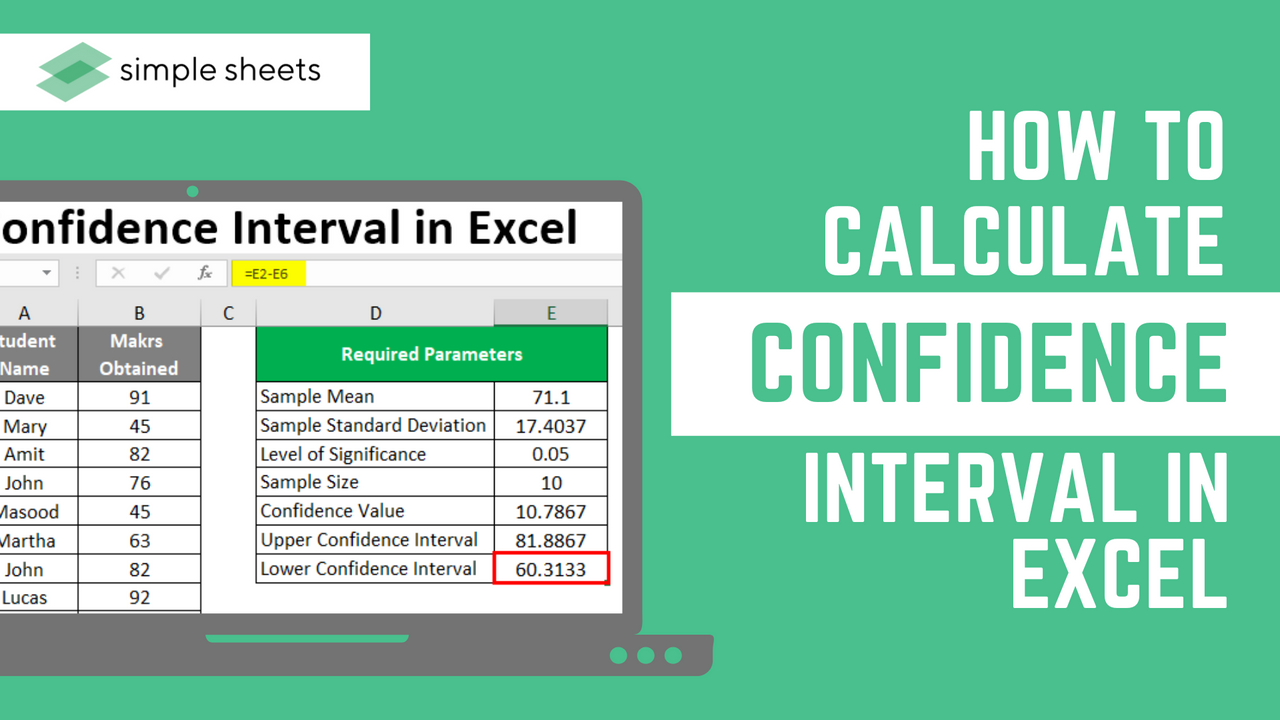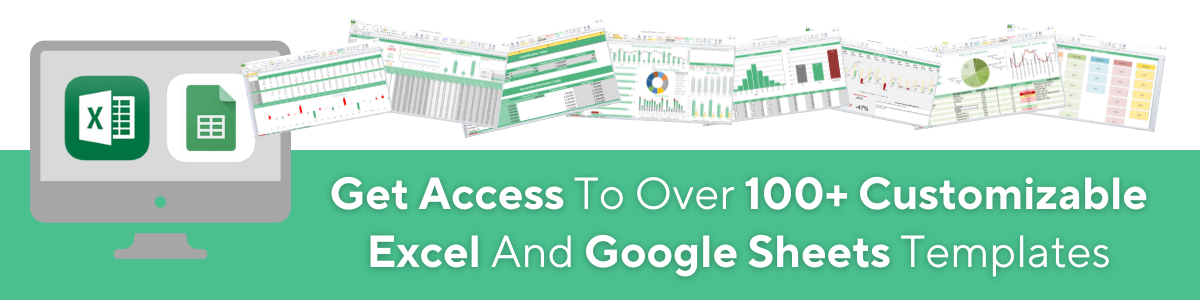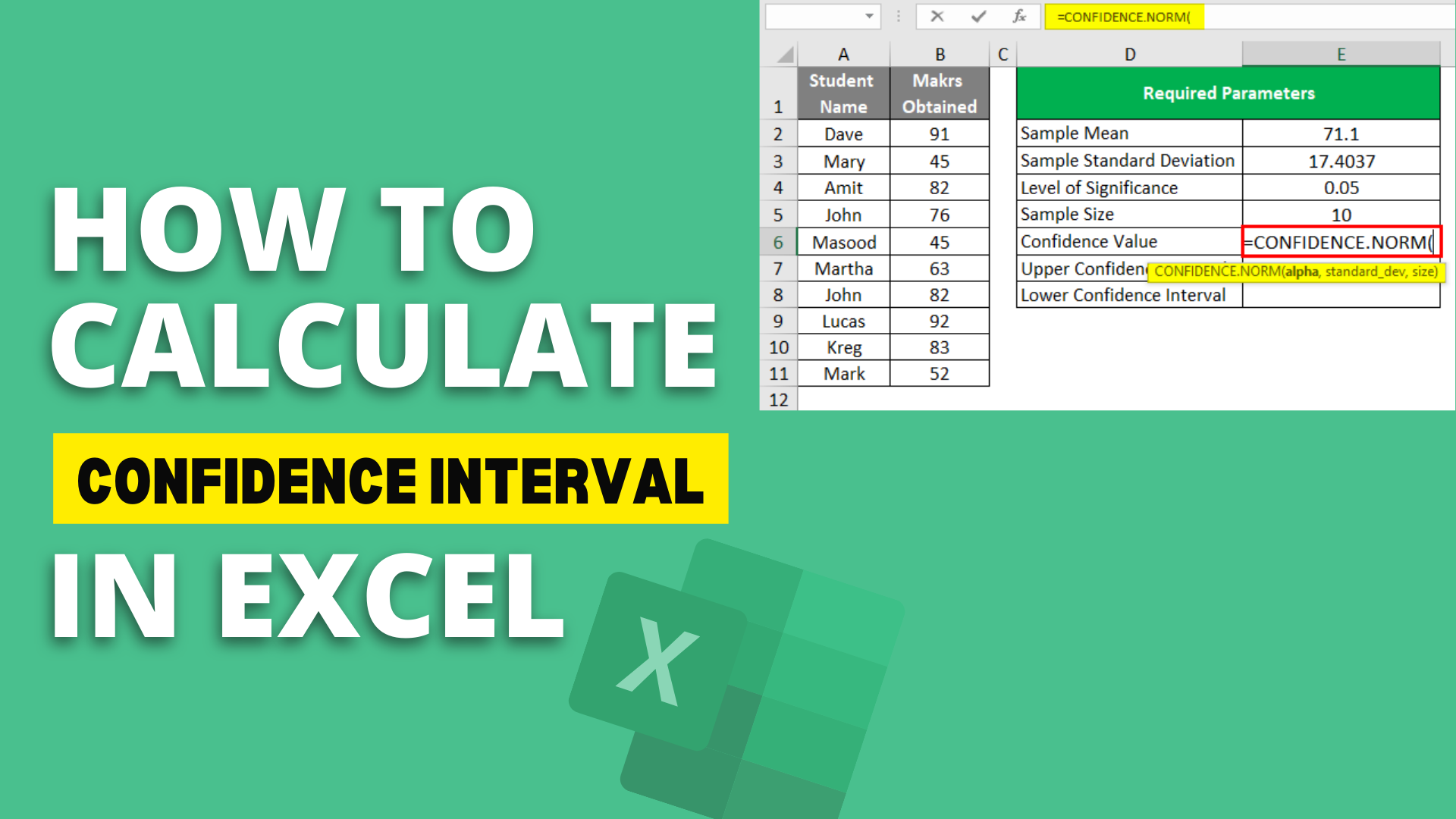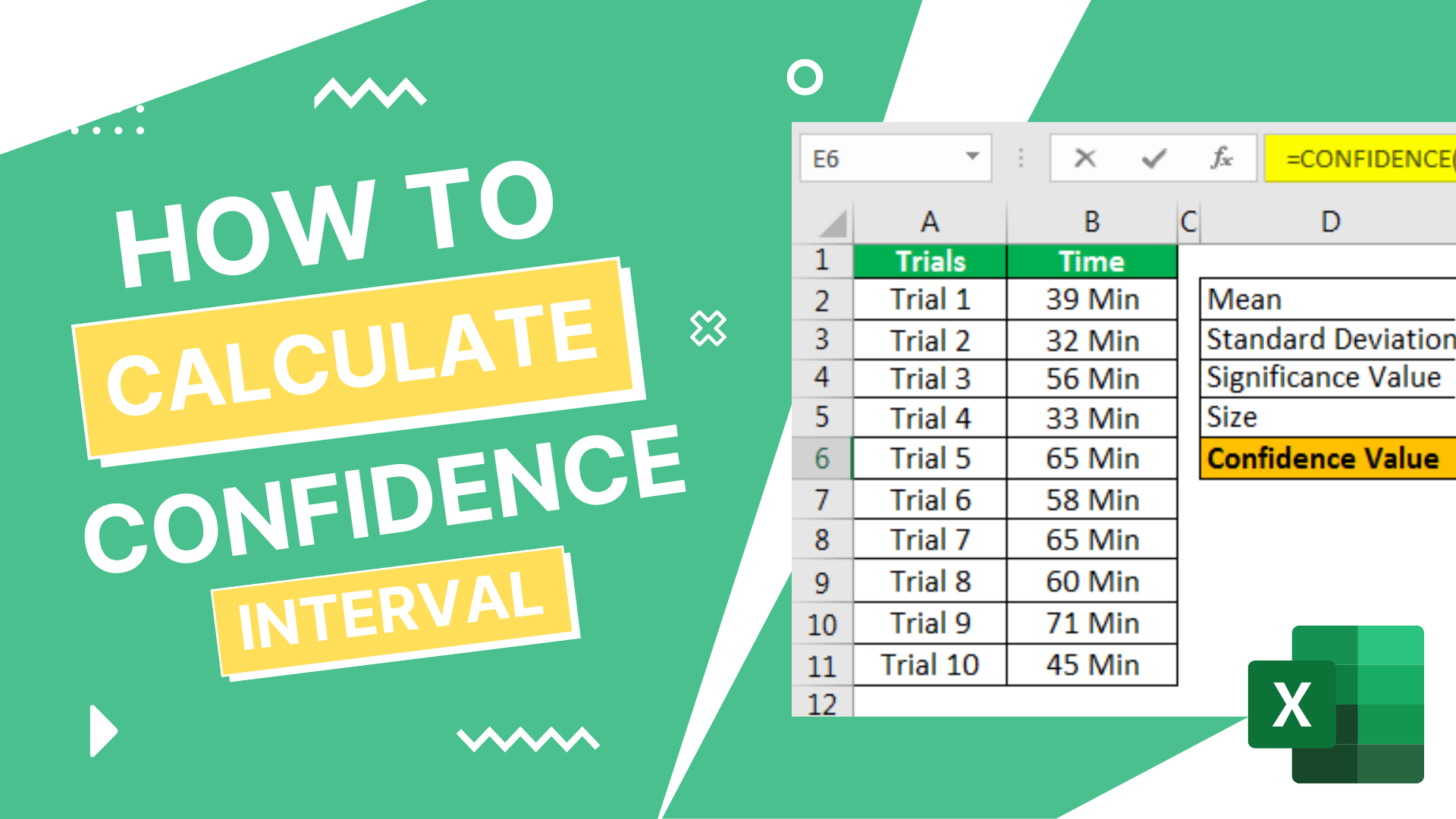# How to Calculate Confidence Interval in Excel: Easy to Follow Steps

Jul 26, 2022

A confidence interval in Excel is calculated using the confidence function. If you are a statistician or financial analyst, you will know how important it is to calculate the confidence interval within Excel. You will also know that learning the margin of error for a sample mean allows for optimal statistical surveys.

This article will discuss the confidence interval in detail and how it is used for data analysis within Excel. Put simply, learning how to calculate confidence intervals in excel is very important.## What Is a Confidence Interval in Excel?

Put simply, a confidence interval in Excel defines a range of values that is located in the center due to where the sample mean is located. The confidence interval within Excel comes from the confidence value statistic.

The confidence function within Excel offers a value that is used to create a confidence interval for a sample mean.

In Excel, the mean value tends to derive from a normal distribution, standard deviation, and sigma.## Why Learn How to Calculate a Confidence Interval in Excel?

### Excel is perfect for statistical computations.

To execute financial and statistical analysis, Excel is the most-used application globally. It is quick to navigate and easy to add statistical functions to projects. Not only that, but Excel offers standard error notifications to let users lower the margin of error.

### Excel is a highly used statistical analysis tool.

Many people rely on Excel for statistical computations. Excel is often seen as the go-to statistical computations. Becoming skilled at Excel allows you to complete many jobs and requirements.

### It is easy to find learning resources.

Due to its popularity, Excel offers quick and easy ways for beginners to learn and improve their skills.

## How to Calculate a Confidence Interval in Excel: A Step-By-Step Guide

### Review the Confidence Function in Your Excel Version

Firstly, it is important to note that if you are using a newer version of Excel, you will not see the confidence function within the list of statistical functions. Instead, it has gotten replaced by CONFIDENCE.NORM and the CONFIDENCE.T functions within Excel.

These two new functions offer better accuracy, which is why they took their place. For this example, I will use CONFIDENCE.NORM function to work out the confidence interval of our sample mean.Now that is out of the way, let's get started! Let's start by assuming the basic test scores from a school offering the below distribution.

38, 15, 14, 22, 24, 21, 18, 12, 13, 14, 30, 25, 20, 15, and 19.

The first thing we need to establish your confidence interval is to work out the standard deviation and mean.

From there, you need to input your significance level.### Calculate the Sample Mean

From there, open your Excel worksheet, and build a column called "Student distribution." Select a blank cell and input the range of values we have highlighted above. These values should cover all the cells from A2 to A16.

Then, from the function tab, select "fx" to choose the mean function. In Excel, this is written as =AVERAGE().

Place your cell reference into the columns so your formula looks like this:

=AVERAGE(A2:A16).

Hit the enter button, and you should see the score of 20 appear.

### Calculate the Standard Deviation

From here, you can now highlight the 17th row to ensure it is easier to see this important information. Next, create a new column using the header "standard deviation."

To work out your sample standard deviation, press the "fx" button. From statistical functions, choose =STDEV.P(). input the values into cells within column B, so the formula looks like this:

=STDEV.P(A2:A16).

Select the enter key to see 6.87992.

### Find the Significance Level and Alpha Value

Okay, let's assume that the significance level we have is 95%. In most instances, the significance level tends to range anywhere from 90 to 99%.

The alpha value refers to the probability value used to determine if a statistical test is without error. To identify the value, solve 1 - alpha, which in this instance is one minus 0.95.

### Calculate the Confidence Value

Now we need to create a third column and name it "confidence value." This column will contain our population sample size, the standard deviation, and our alpha value. To work out the confidence rate, implement this formula:

=CONFIDENCE.NORM(alpha,standard_dev,size).

### Calculate the Interval of Confidence

Don't forget that the confidence interval refers to the range of cells with the sample mean in the center. To establish the accurate computation of our confidence level, we must subtract our confidence value from the sample mean and include the confidence value to increase our confidence limit.

## How to Calculate a Confidence Interval in Excel at Once

If you already know your standard deviation, alpha value, and sample mean value, you can calculate your confidence interval directly in Excel using the Excel confidence function.

However, you must have all the components, so ensure that all of the data range you enter into the confidence interval formula is right, to get an accurate confidence interval.## Benefits of Calculating a Confidence Interval in Excel

### Excel offers a more simplified process.

Unlike a normal workbook, you have the power to build a concise statistical computation within an Excel sheet.

Excel offers features that ensure building statistical models is extremely simple.

### It is time-saving.

Discovering statistical functions and calculating values in Excel is very easy once you get started. You can save a lot of time on computing complex data, as Excel does this automatically, so long as you input the correct values.

### Microsoft Excel is accessible.

One simple factor that ensures Excel is good is because of how accessible it is. You can access Excel for an affordable yearly cost and use it to complete many different tasks.

## Related Articles

How to Delete Sheets in Excel: Deleting Multiple Sheets at Once

Microsoft Excel Certification: How to Become a Professional

Free Microsoft Excel Tutorial For Beginners

Lorem ipsum dolor sit amet, consectetur adipiscing elit. Cras sed sapien quam. Sed dapibus est id enim facilisis, at posuere turpis adipiscing. Quisque sit amet dui dui.